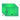# Rectangular Volume and Area Calculator

Width (W)

Length (L)

Distance (D)

Select Unit### How To Calculate Rectangle Volume?

Volume = width * lenght * distance ,
ex : width = 15m, lenght = 20, distance = 25
Volume = 15 * 20 * 25 = 7500m3

### Rectangle Volume Info

The volume of a Rectangle is generally known to be the capacity of the Rectangle. Volume is usually quantified numerically using the SI derived unit, the cubic meter.

### How To Calculate Rectangle area?

Total Area = 2 * (width * distance + width * lenght + distance * lenght) .
ex : width = 10, lenght = 30, distance = 20.
Area = 2* ( 10 * 20 + 20 * 30 + 20 * 30 ) = 2200 m2.